Test: Continuity and Differentiability- Assertion & Reason Type Questions

# Test: Continuity and Differentiability- Assertion & Reason Type Questions

Test Description

## 8 Questions MCQ Test Mathematics (Maths) Class 12 | Test: Continuity and Differentiability- Assertion & Reason Type Questions

Test: Continuity and Differentiability- Assertion & Reason Type Questions for Commerce 2023 is part of Mathematics (Maths) Class 12 preparation. The Test: Continuity and Differentiability- Assertion & Reason Type Questions questions and answers have been prepared according to the Commerce exam syllabus.The Test: Continuity and Differentiability- Assertion & Reason Type Questions MCQs are made for Commerce 2023 Exam. Find important definitions, questions, notes, meanings, examples, exercises, MCQs and online tests for Test: Continuity and Differentiability- Assertion & Reason Type Questions below.
Solutions of Test: Continuity and Differentiability- Assertion & Reason Type Questions questions in English are available as part of our Mathematics (Maths) Class 12 for Commerce & Test: Continuity and Differentiability- Assertion & Reason Type Questions solutions in Hindi for Mathematics (Maths) Class 12 course. Download more important topics, notes, lectures and mock test series for Commerce Exam by signing up for free. Attempt Test: Continuity and Differentiability- Assertion & Reason Type Questions | 8 questions in 16 minutes | Mock test for Commerce preparation | Free important questions MCQ to study Mathematics (Maths) Class 12 for Commerce Exam | Download free PDF with solutions
 1 Crore+ students have signed up on EduRev. Have you?
Test: Continuity and Differentiability- Assertion & Reason Type Questions - Question 1

### Directions: In the following questions, A statement of Assertion (A) is followed by a statement of Reason (R). Mark the correct choice as.Assertion: If y = sin-1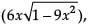then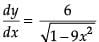Reason: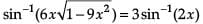Detailed Solution for Test: Continuity and Differentiability- Assertion & Reason Type Questions - Question 1 put 3x = sin θ or θ = sin-1 3x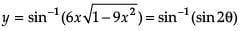= 2θ

= 2 sin-1 3x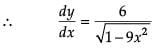A is true. R is false.

Test: Continuity and Differentiability- Assertion & Reason Type Questions - Question 2

### Directions: In the following questions, A statement of Assertion (A) is followed by a statement of Reason (R). Mark the correct choice as.Assertion : |sin x| is continuous for all x ∈ R.Reason : sin x and |x| are continuous in R.

Detailed Solution for Test: Continuity and Differentiability- Assertion & Reason Type Questions - Question 2 sin x and |x| are continuous in R. hence R is true.

Consider the functions f(x) = sin x and g(x) = |x| both of which are continuous in R.

gof(x) = g(f(x)) = g(sin x) = |sin x |.

Since f(x) and g(x) are continuous in R, gof(x) is also continuous in R.

Hence A is true.

R is the correct explanation of A.

Test: Continuity and Differentiability- Assertion & Reason Type Questions - Question 3

### Directions: In the following questions, A statement of Assertion (A) is followed by a statement of Reason (R). Mark the correct choice as.Assertion : A continuous function is always differentiable.Reason : A differentiable function is always continuous.

Detailed Solution for Test: Continuity and Differentiability- Assertion & Reason Type Questions - Question 3 The function f(x) is differentiable at x = a, if it is continuous at x = a and LHD = RHD at x = a.

A differentiable function is always continuous. Hence R is true.

A continuous function need not be always differentiable.

For example, |x| is continuous at x = 0, but not differentiable at x = 0.

Hence A is false.

Test: Continuity and Differentiability- Assertion & Reason Type Questions - Question 4

Directions: In the following questions, A statement of Assertion (A) is followed by a statement of Reason (R). Mark the correct choice as.

Assertion : f(x) = tan2 x is continuous at x = π/2

Reason : g(x) = x2 is continuous at x = π/2.

Detailed Solution for Test: Continuity and Differentiability- Assertion & Reason Type Questions - Question 4 g(x) = x2 is a polynomial function. It is continuous for all x ∈ R.

Hence R is true.

f(x) = tan2 x is not defined when x = π/2.

Therefore f(π/2) does not exist and hence f(x) is not continuous at x = π/2.

A is false.

Test: Continuity and Differentiability- Assertion & Reason Type Questions - Question 5

Directions: In the following questions, A statement of Assertion (A) is followed by a statement of Reason (R). Mark the correct choice as.

Assertion : f(x) = [x] is not differentiable at x = 2.

Reason : f(x) = [x] is not continuous at x = 2.

Detailed Solution for Test: Continuity and Differentiability- Assertion & Reason Type Questions - Question 5 f(x) = [x] is not continuous when x is an integer.

So f(x) is not continuous at x = 2. Hence R is true.

A differentiable function is always continuous.

Since f(x) = [x] is not continuous at x = 2, it is also not differentiable at x = 2.

Hence A is true.

R is the correct explanation of A.

Test: Continuity and Differentiability- Assertion & Reason Type Questions - Question 6

Directions: In the following questions, A statement of Assertion (A) is followed by a statement of Reason (R). Mark the correct choice as.

Consider the function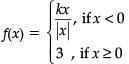which is continuous at x = 0.

Assertion (A): The value of k is – 3.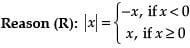Detailed Solution for Test: Continuity and Differentiability- Assertion & Reason Type Questions - Question 6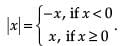This is the definition for modulus function and hence true.

Hence R is true.

Since f is continuous at x = 0,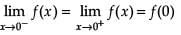Here f(0) = 3,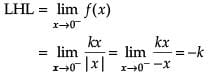∴ -k = 3 or k = -3

Hence A is true.

R is the correct explanation of A.

Test: Continuity and Differentiability- Assertion & Reason Type Questions - Question 7

Directions: In the following questions, A statement of Assertion (A) is followed by a statement of Reason (R). Mark the correct choice as.

Assertion (A): |sin x| is continuous at x = 0.

Reason (R): |sin x| is differentiable at x = 0.

Detailed Solution for Test: Continuity and Differentiability- Assertion & Reason Type Questions - Question 7 Since sin x and |x| are continuous functions in R, |sin x| is continuous at x = 0.

Hence A is true.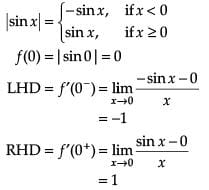At x = 0, LHD ≠ RHD.

So f(x) is not differentiable at x = 0.

Hence R is false.

Test: Continuity and Differentiability- Assertion & Reason Type Questions - Question 8

Directions: In the following questions, A statement of Assertion (A) is followed by a statement of Reason (R). Mark the correct choice as.

Consider the function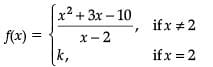which is continuous at x = 2.

Assertion (A): The value of k is 0.

Reason (R): f(x) is continuous at x = a, if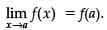Detailed Solution for Test: Continuity and Differentiability- Assertion & Reason Type Questions - Question 8 f(x) is continuous at x = a,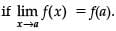∴ R is true.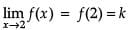∴ k = 7

Hence A is false.

## Mathematics (Maths) Class 12

209 videos|218 docs|139 tests
Information about Test: Continuity and Differentiability- Assertion & Reason Type Questions Page
In this test you can find the Exam questions for Test: Continuity and Differentiability- Assertion & Reason Type Questions solved & explained in the simplest way possible. Besides giving Questions and answers for Test: Continuity and Differentiability- Assertion & Reason Type Questions, EduRev gives you an ample number of Online tests for practice

## Mathematics (Maths) Class 12

209 videos|218 docs|139 tests(Scan QR code)